Owen LeeKotlin 修炼手册（8）杂项知识点

1. 类型别名
2. 位运算
3. 运算符重载

#### 类型别名

// 缩短集合类型
typealias NodeSet = Set<Network.Node>
typealias FileTable<T> = MutableMap<T, MutableList<File>>

// 缩短内部类
class A{
inner class Inner
}
typealias AInner = A.Inner

Kotlin 中也支持对 Lambda 表达式的类型指定别名：

typealias Predicate<T> = (T) -> Boolean

val p: Predicate<String> = {it.length > 4}
println(arrayOf("Kotlin","Java","Go","Python").filter(p))

// 运行结果
["Kotlin", "Python"]

#### 位运算

1. 按位与、按位或、按位异或
println(5 and 9) // 输出1
println(5 or 9)  // 输出13
println(5 xor 9) // 输出12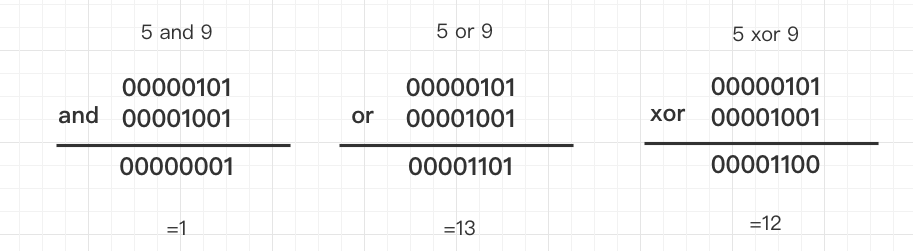1. 按位非
println(5.inv())    // 输出-6
println((-5).inv()) // 输出4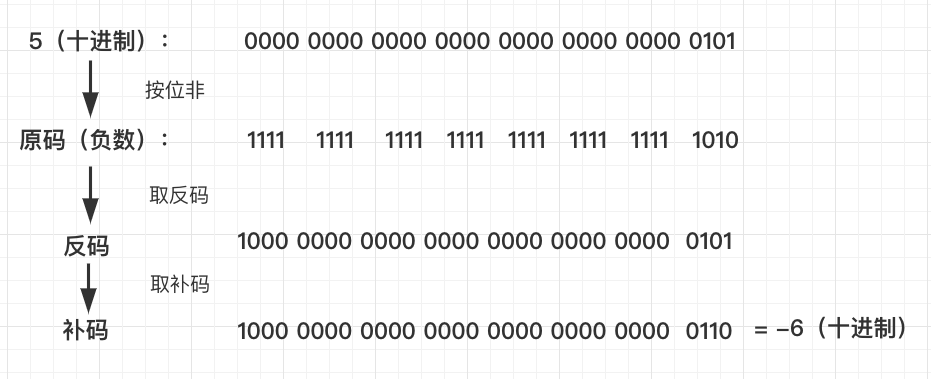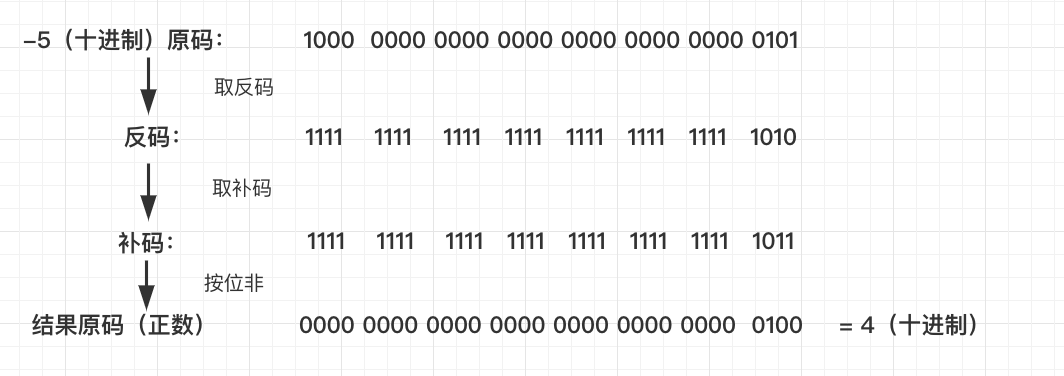1. 左移运算符
println(5 shl 2)    // 输出20
println(-5 shl 2)   // 输出-20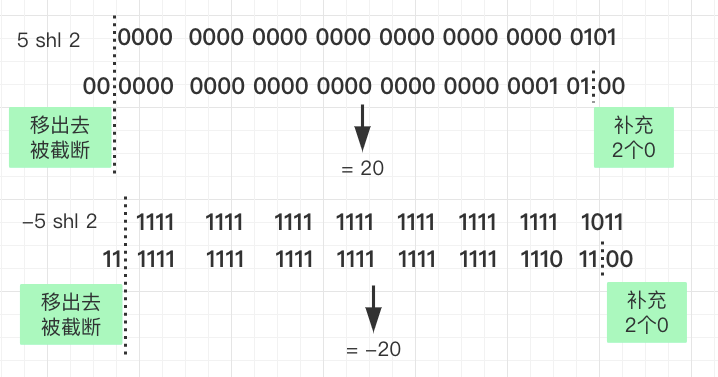1. 右移运算符、无符号右移运算符
println(-5 shr 2)    // 输出-2
println(-5 ushr 2)   // 输出1073741822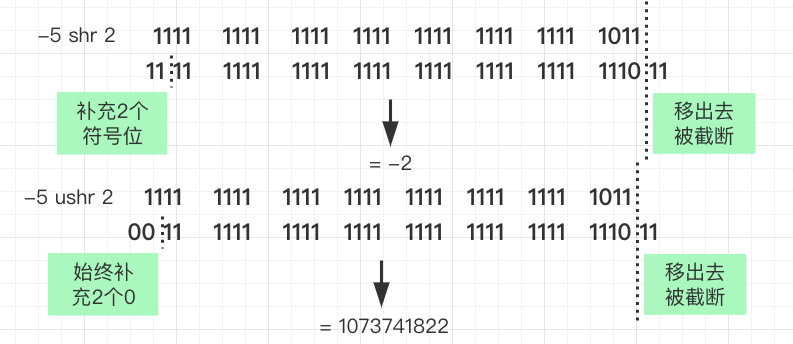#### 运算符重载

+a a.unaryPlus()
-a a.unaryMinus()
!a a.not()

a++ a.inc()
a– a.dec()

a + b a.plus(b)
a - b a.minus(b)
a * b a.times(b)
a / b a.dev(b)
a % b a.rem(b)
a .. b a.rangeTo(b)

in 和 !in 运算符

a in b b.contains(a)
a !in b !b.contains(a)

a[i] a.get(i)
a[i,j] a.get(i,j)
a[i1,..,im] a.get(i1,..,im)
a[i] = b a.set(i,b)
a[i,j] = b a.get(i,j,b)
a[i1,..,im] = b a.get(i1,..,im,b)

a() a.invoke()
a(b) a.invoke(b)
a(b1, b2) a.invoke(b1, b2)

a += b a.plusAssign(b)
a -= b a.minusAssign(b)
a *= b a.timesAssign(b)
a /= b a.devAssign(b)
a %= b a.remAssign(b)

a == b a?.equals(b) ?: (b===null)
a != b !(a?.equals(b) ?: (b===null))

a > b a.compareTo(b) > 0
a >= b a.compareTo(b) >= 0
a < b a.compareTo(b) < 0
a <= b a.compareTo(b) <= 0

data class Point(val x: Int, val y: Int) {
// 重载 minus 函数，就可以使用 p1 - p2
operator fun minus(target: Point): Double {
return Math.hypot((x - target.x).toDouble(), (y - target.y).toDouble())
}

// 重载 times 函数，就可以使用 p1 * p2
operator fun times(target: Point): Int {
return Math.abs(x - target.x) * Math.abs(y - target.y)
}
}

fun main() {
val p1 = Point(0,0)
val p2 = Point(3,4)
println("两个点的距离：${p1 - p2}") println("两个点围成的举行面积：${p1 * p2}")
}

// 运行结果